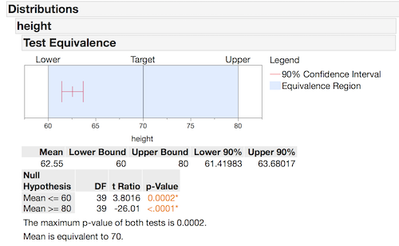Our World Statistics Day conversations have been a great reminder of how much statistics can inform our lives. Do you have an example of how statistics has made a difference in your life? Share your story with the Community!
Choose Language Hide Translation Bar
Highlighted

## Equivalence Test when Variances are Unequal

Is there a built in way (or straightforward way to script) to test for equivalence of means with a procedure similar to TOST when the variances of groups are unequal (significant result from the appropriate test under JMP's "Unequal Variances" report).

I am assuming that since the TOST procedure that is part of JMP's "Equivalence Test" option in the Fit Y by X platform relies on two t-tests, the variance is pooled by default.

Thank you.

1 ACCEPTED SOLUTION

Accepted Solutions
Highlighted

## Re: Equivalence Test when Variances are Unequal

Its not clear from your post exactly how you're going to use the test for equivalence.  In TOST I set an upper and lower limit, and then I put the 95% CI on the limits and make sure that my mean is between the confidence intervals. In the distribution platform you get a result like this (below) and there is no pooling of variance. Its just the variance of the univariate statistic.This graph has the upper and lower equivalence limits (defined by me as the difference to consider negligible) with the variable's mean bordered by a one side 95% CI on each side (or 90% CI of the mean, same thing). If the CI crosses the equivalence boundary, then it is not equivalent.  The more precise a measurement system is the closer I can get to the boundary with out crossing it.

I can use the Fit Y by X platform to calculate 90% CIs for a whole group of variables (by JSL) the grab the results table as a data table (by JSL) and use the Graph Builder to make a similar plot (save the graph script and use that too.)   Since equivalence testing compares each group mean and its variance with the equivalence limits, there is no pooling of variance. Variance is pooled (or not pooled) when I'm comparing the group means to each other like in a t-test.

JMP Systems Engineer, Pharm and BioPharm Sciences
4 REPLIES 4
Highlighted

## Re: Equivalence Test when Variances are Unequal

Its not clear from your post exactly how you're going to use the test for equivalence.  In TOST I set an upper and lower limit, and then I put the 95% CI on the limits and make sure that my mean is between the confidence intervals. In the distribution platform you get a result like this (below) and there is no pooling of variance. Its just the variance of the univariate statistic.This graph has the upper and lower equivalence limits (defined by me as the difference to consider negligible) with the variable's mean bordered by a one side 95% CI on each side (or 90% CI of the mean, same thing). If the CI crosses the equivalence boundary, then it is not equivalent.  The more precise a measurement system is the closer I can get to the boundary with out crossing it.

I can use the Fit Y by X platform to calculate 90% CIs for a whole group of variables (by JSL) the grab the results table as a data table (by JSL) and use the Graph Builder to make a similar plot (save the graph script and use that too.)   Since equivalence testing compares each group mean and its variance with the equivalence limits, there is no pooling of variance. Variance is pooled (or not pooled) when I'm comparing the group means to each other like in a t-test.

JMP Systems Engineer, Pharm and BioPharm Sciences
Highlighted

## Re: Equivalence Test when Variances are Unequal

My apologies if I'm missing something here, as I had the same question about performing the TOST test to compare two groups with unequal variances and I'm not following the reply.  Per the description of JMP's equivalence TOST test, "Two one-sided pooled-variance t tests are constructed for the null hypotheses that the true difference exceeds the threshold values."  So, all I wish to do is to perform exactly the same test, but using t-tests with unequal variances.  The confidence intervals for the difference in means between the two groups are slightly different when they are determined using pooled variances or when assuming unequal variances.  So I would like to perform the TOST test using the appropriate confidence intervals.

Highlighted

## Re: Equivalence Test when Variances are Unequal

Is the difference in the variances significant?

Learn it once, use it forever!
Highlighted

## Re: Equivalence Test when Variances are Unequal

It's often not huge, but in some cases, it can push the TOST results from below significance at 0.05 to above. I realize that aspects of the TOST approach, such as setting the equivalence threshold, can be a bit arbitrary, so I'm possibly nit-picking a detail that has less effect than other choices that I might make.  But, I was just hoping to set up a fixed approach for some data, in which the variances of the groups would first be compared and then the TOST would be performed using either the pooled variance test or the test that does not assume equal variances, whichever is appropriate.

I set up a script in which the 90% confidence interval is determined for the difference between the groups when we don't assume equal variances.  That confidence interval can then be compared to the interval determined using the desired equivalence threshold, giving a yes/no answer on the equivalence question.  So, I could live with this, if I must.  The piece that I would like to improve is the determination of the maximum p-value, as well as the nice graphical representation of the results, both of which the built-in JMP equivalence test provides.

Article Labels

There are no labels assigned to this post.